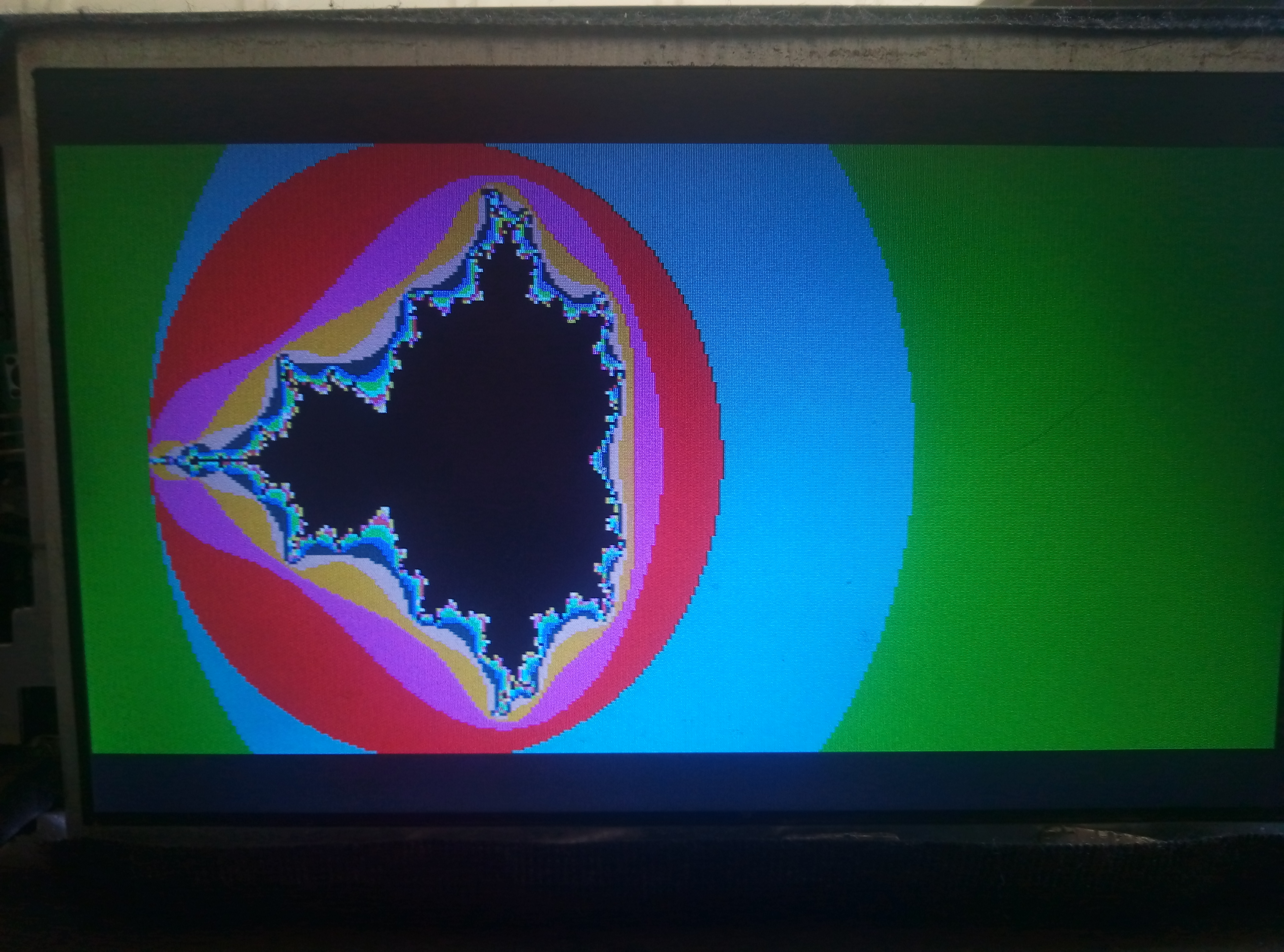# Θεόδουλος Λιοντάκης

## Δάσκαλος Πληροφορικής ΠΕ86

### Στατιστικά

Εμφανίσεις Άρθρων
15949

Tiny Basic for Lion is compatible with Palo Alto Tiny Basic but has a lot of new commands and fixed point arithmetic operation support added.

Original Palo Alto Language pdf

Fixed point arithmetic allows decimals but because in Lion TB it uses only 32bits it has small  precision (3 digits).

LTB also has the following math functions: COS, SIN, INT, ROUND, SQRT, PI   ex. Y=COS(X)  x from -pi to pi

Lion TB has doubled the possible  variables because it distinguishes between small and capital letters so a..z and A..Z variables are available. Commands are recognised in both small and capitals.

Lion TB supports FAT16 file system.  It knows 3 kinds of files .BAS .BIN and .SCR

Saves a basic file using SAVE, ex. SAVE "TEST2" again the extension .BAS is omitted

With DIR command it displays the current directory

CD command changes directory   examples   CD "VECTOR"      CD ..

Delete a file using DELETE command, example  DELETE "TEST2.BAS" extension must be included

Load a binary file using the LCODE filename,address command, example: LCODE "MANDEL",20000  loads file MANDEL.BIN starting at address 20000   Then you can execute the code you loaded at the address 20000 using command RCODE 20000

A special variable named BTOP contains the first available address after the space currently used by basic, so a usual way to execute relocateable binary code (as produced by Lion java compiler)  and then return to basic would be   LCODE "PROG1",BTOP  and then RCODE BTOP

A binary file can be loaded from the serial port using the command GCODE address,length

Screen and graphics

CLS command clears the screen

CLSP command disables all sprites and hides them from the screen

POS Y,X sets the printing position, X=0..79 Y=0..29 for mode 0 and X=0..52 Y=0..24 for mode 1

MODE commands selects 0 or 1 graphics modes

Mode 0, text optimized, resolution 640x240, 80x30 chars, 16 colors restricted to 2 per char

Mode 1, graphics optimized, resolution 320x200, 53x25 chars, 16 colors no restriction

In mode 0 command SCREEN N sets both foreground and background color with the formula N=16*foreground + background, background and foreground are numbers 0..15 that correspond to colors 0 to 15

Also in mode 0 the command COLOR X,Y,N sets the color of individual caracter at X,Y to N

In mode 1 color is changed with 2 commands BCOLOR and FCOLOR

BCOLOR N where N=0 to 15, sets background color  and FCOLOR N where N=0 to 15, sets foreground color

In both modes graphics are drawn using the commands PMODE, PLOT, LINE, CIRCLE

PMODE n where n=0  or 1 sets the way the other graphic commands draw, when 0 they use foreground color, when 1 they use the background

PLOT X,Y plots a point at X,Y

LINE X1,Y1,X2,Y2  draws a line from x1,y1 to x2,y2

CIRCLE X1,Y1,R draws a circle with center x1,y1 and radius R

Sound

Lion has 3 sound channels + noise

BEEP F,T,C  makes a sound of frequency F, duration T= 0 to 7, Channel C=0..2

To add noise OUT 11,1 to remove noise OUT 11,0

To change volume OUT 25+C,V   where C=0..3 and V=0..255

Sprites

Sprite TB commands are also to be implemented and currently sprites can be controlled with the OUT OUTB and INP INPB commands

Input

Function WAITK waits for a keypress and returns the ascii code of the key pressed

Function KEY returns the ascii code of a pressed key but doesn't wait for a key press

Functions JOY1  and JOY2 return the joystick status (binary: 0 0 0 Right Left Up Down Button)

10 J=JOY1

20 IF J=16 PRINT "RIGHT"

30 IF J=1 PRINT "FIRE BUTTON PRESSED"

40 K=WAITK

50 PRINT K

Misc.

Function TIMER returns a value in milliseconds that resets every 65536ms

Command SLIST lists basic program to serial port

program example:

5 MODE 1
10 BCOLOR 0 ; CLS
300 E=-1.3
310 FOR Y=0 TO 199
320 D=-2.4
330 FOR X=0 TO 299
340 Z=0; I=0; A=1
350 REM LOOP
360 S=(Z*Z)-(I*I)+D
370 R=(2*I*Z)+E
380 Z=S
390 I=R
400 A=A+1
405 L=Z*Z+I*I
410 IF ((A<16)*(L<4)) GOTO 360
500 FCOLOR A
510 PLOT X,Y
520 D=D+0.0215
530 NEXT X
540 E=E+0.013
550 NEXT Y
560 FCOLOR 15
570 K=WAITK

program output: